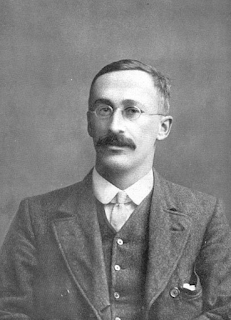# Data Science Simplified

Learning the Machine Learning, in a Human-friendly Way

### A Beginner's Guide to t-tests: Real-life Applications of t-test: One-Sample, Two Sample and Paired Sample t-test

The t-test was developed by William Sealy Gosset, an English statistician and a beer brewer.Image source

William Sealy Gosset used this test to produce consistent and also high-quality beer. He published this test in a paper under the pen-name "Student". Hence, this test is also called the Student's t-test.

Three types of t-test:

A) One-sample t-test

Suppose you represent a testing agency. The government wants to know whether the average weight of a certain species of animal is 10 kg. To test this, you take a random sample (of let us say 10 animals) and use the one-sample t-test to check whether the sample mean is 10 kg or is it statistically different from 10 kg.

B) Two-sample t-test

You own a shop. There are two major consumer groups: i) those who visit your shop by car and ii) those on a motorbike. You want to test - is there any difference in spending between these two consumer groups? For this purpose, you use a two-sample t-test.

The two-sample t-test is also used to analyse the results from A/B testing.

What if there are more than two groups?
Then, we have to perform pair-wise two-sample t-tests, which is cumbersome.
A better approach is to perform ANOVA (Analysis of Variance).

What if the variances of the two samples are different?
In that case, we have to use Welch's t-test, also called the unequal variances t-test.

C) Paired samples t-test

You are a trainer. You trained 10 students. You want to know if there was any improvement in students' knowledge level due to training. To compare, pre-training scores with post-training scores, you use paired samples t-test.

This test may appear similar to a two-sample t-test but there is a vital difference. In paired samples t-test, subjects (in this example, students) are the same. In paired samples t-test, we compare the same subject (students in this example) using some measures (e.g. scores in exams) before and after the interventions (i.e. training in this example).

Assumptions of t-test
• Normally distributed data
• If this assumption is not valid, then we have to use non-parametric tests
• Samples are drawn randomly from the population
• Homogeneity of variance (variability of data in each group is similar)
• If this assumption is not fulfilled, then we can use Welch's t-test, also called the unequal variances t-test.